## WitCH 8: Oblique Reasoning

A reminder, WitCH 2, WitCH 3 and WitCH 7 are also open for business. Our new WitCH comes courtesy of John the Merciless. Once again, it is from Cambridge’s text Specialist Mathematics VCE Units 3 & 4 (2019). The text provides a general definition and some instruction, followed by a number of examples, one of which we have included below. Have fun.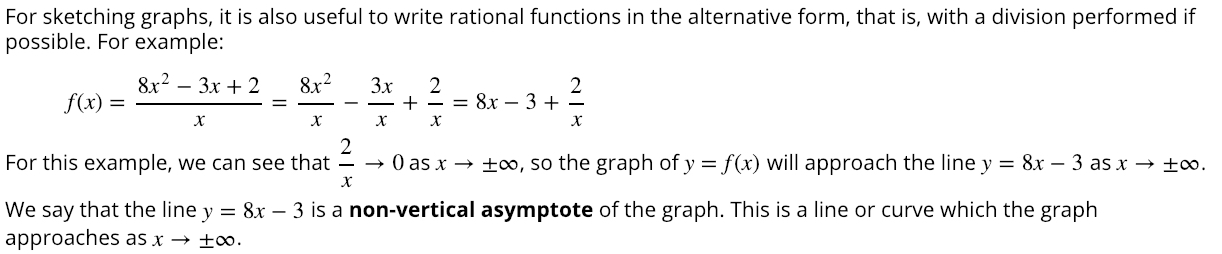Update

With John the Impatient’s permission, I’ve removed John’s comments for now, to create a clean slate. It’s up for other readers to do the work here, and (the royal) we are prepared to wait (as is the continuing case for WitCh 2 and Witch 3).

This WitCH is probably difficult for a Specialist teacher (and much more so for other teachers). But it is also important: the instruction and the example, and the subsequent exercises, are deeply flawed. (If anybody can confirm that  exercise 6G 17(f) exists in a current electronic or hard copy version, please indicate so in the comments.)

Update (05/02/20)

It is obviously long, long past time to sort out this godawful mess. We apologise to all those industrious commenters, who nailed the essential wrongness, and whose hard work was left hanging. This will also be a very long update; you should pour yourself a stiff drink, grab the bottle and get comfy. For the benefit of the Twitter addicts, however, who now find it difficult to concentrate for more than two paragraphs, here’s the punchline:

Cambridge‘s notion of “non-vertical asymptote” is so vague, falling so short of a proper definition, it is close to meaningless and it is pointless. This leads to the claimin Example 31 being flawed in three distinct ways. In particular, it follows from Cambridge that the “curve”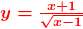is a “non-vertical asymptote” to the function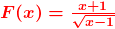.

The source of Cambridge‘s confusion is also easy to state:

More complicated asymptotes cannot be defined, interpreted or computed in the manner possible for simpler asymptotes.

Unfortunately, the discussion in Cambridge is so sparse and so far from coherent that directly critiquing the excerpt above would probably be incomprehensible. So, we’ll first try to make very careful sense of “non-vertical asymptotes”, taking some minor whacks at Cambridge along the way. Then, with that sense as foundation, it will be easy work to hammer the excerpt above. To simplify the discussion, we’ll only consider the asymptotes of a functionas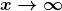. Obviously, the case where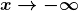can be handled in like manner, and vertical asymptotes are pretty straightforward.

OK, take a swig and let’s go.

1. Horizontal Asymptotes

We’ll begin with the familiar and demon-free case, for asymptotes as horizontal lines. Consider, for example, the functionWe can write, noting the second term is tiny when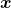huge. So, we declare thathas the horizontal asymptote.

Formally, the asymptote in this example is captured with limits. The underlying functional behaviour is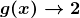or, more officially,. The limit formalism is of no benefit here, however, and is merely likely to confuse. The informal manner of thinking about and writing limits, as is standard in schools, suffices for the understanding of and computation of horizontal asymptotes.

Note also that horizontal asymptotes can easily be spotted with essentially no calculation. Consider the function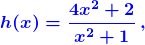which is Example 29 in Section 6G of Cambridge. Here, it is only the highest powers, theterms in the numerator and denominator, that matter; thes cancel, and so the function has horizontal asymptote. This simplification also applies to suitable rooty (algebraic) functions. The function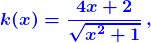for example, also has horizontal asymptote, for the same reason.

2. Linear Asymptotes

We’ll now consider general linear asymptotes, which are still reasonably straight-forward (and which include horizontal asymptotes as a special case). There are, however, two demons lurking, one conceptual and one computational.

Cambridge begins with the example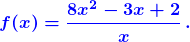The function can be rewritten as, and it seems simple enough: a similar “whenis huge, the leftovers are tiny” intuition leads us to declare that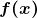has the linear asymptote. The trouble is that the example, and the discussion that follows, are too simple, so that the demons remain hidden.

3. A Conceptual Demon

How do we formally (or semi-formally) express that a function has a linear asymptote? For the example above, Cambridge writes “f(x) will approach the line y = 8x – 3”. This is natural, intuitive and sufficiently clear. It is considering the asymptote to be a geometric object, as the line, which is fine at the school level.

What, however, if we want to think of the asymptote as the function? The problem is that bothand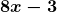are zooming off to infinity, which means that writing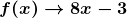, or anything similar, is essentially meaningless. Those arrows are shorthand for limits and, fundamentally, the limit of a function must be a number, not another function. (For vertical asymptotes we consider infinity to be an honorary number, which may seem dodgy, but which can be justified.)

The obvious way around this problem is simply to ignore it. As long as we stick to asymptotes of reasonably simple functions – rational functions and carefully chosen others – Cambridge’s intuitive approach is fine. But in the, um, unlikely event that our situation is not so simple, then we have to carefully consider the limiting behaviour of functions. So, we’ll continue.

Suppose that the functionappears to have the linear functionas asymptote. To capture this idea, the simple trick is to consider the difference of the two functions. Ifis huge then this difference should be tiny, and sobeing the asymptote tois captured by the limit statementAgain, none of this is really necessary for capturing linear asymptotes of simple functions. But it is necessary, at least as underlying guidance, if we wish to consider less simple functions and/or more general asymptotes.

WARNING: It may seem as if our boxed definition of linear asymptotewould work just as well fornon-linear, but there is a trap. There is another, third demon to deal with. Before that, however, our second demon.

4. A Computational Demon

It is very import to understand that the simple, “highest powers” trick we indicated above for spotting horizontal asymptotes does not work for general linear asymptotes. Cambridge‘s introductory exampleillustrates the issue, albeit poorly and with no subsequent examples. For a better illustration, we have Exercise 12 in Section 6G, which presents us with the function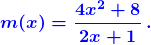Here, it would be invalid to divide theby the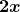and then declare the linear asymptote to be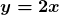. The problem is thein the denominator has an effect, and we are forced to perform long division or something similar to determine that effect. So,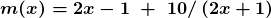, and then it is clear that the linear asymptote is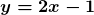.

All that is fine, as far as it goes. Cambridge routinely performs long division to correctly determine linear asymptotes (including horizontal asymptotes, for which the simpler highest powers trick would have sufficed). What, however, if the function is not rational? Consider, for example, Example 31 above. Can we safely ignore the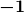in the denominator of the function, as Cambridge has done? And, if so, why?

In order to stay in the linear world for now, we’ll leave Example 31 and instead consider two other examples: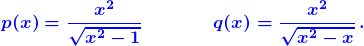So, are we permitted to ignore theand the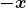in the denominators ofand? It turns out that the answers are “Yes” and “No”: the functionhas linear asymptote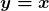, buthas linear asymptote.

The behaviour ofandis, of course, anything but obvious. In particular, the “highest powers” and long division tricks are of no assistance here. Moreover, similar difficulties arise with the analysis of non-horizontal asymptotes of pretty much all rooty functions. It is essentially impossible for a Victorian school text to cover non-horizontal asymptotes of non-rational functions. The text must either work very hard, or it must cheat very hard; Cambridge takes the latter approach.

5. A Nonlinear Demon

Almost there. We have non-linear “asymptotes” left to consider, which are fundamentally demonic. This is demonstrated by Cambridge‘s very first example, Example 26, which considers the functionDividing through bygives, after which Cambridge baldly declares “The non-vertical asymptote has equation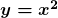“. But why, exactly? All we have to go on is Cambridge‘s intuitive approach toabove. Intuitively, the function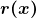approaches the parabola. That’s fine, and we can formalise it by setting. Then guided by our boxed definition above, we can write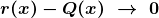, and we seem to have our asymptote.

The problem is that there are a zillion functions that will fit into that blue box. It is also true, for example, that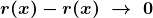. This means thatitself just as good an asymptote as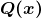. So, yes, one can reasonably declare thatis asymptotic to, but we cannot declareto be THE asymptote to.

Of course we want asymptotes to be unique, so what do we do? There are two ways out of this mess, the first solution being to restrict the type of function that we’ll permit to be an asymptote. That’s intrinsically what we did when considering horizontal and linear asymptotes, and it is exactly why the multiple asymptote problem didn’t arise in those contexts: linear functions only had to compete with other linear functions. Now, for general rational functions, we must broaden the notion of asymptote, but it’s critical to not overdo it: we now permit polynomial asymptotes, but nothing more general. Then, every rational function will have a unique polynomial (possibly linear, possibly horizontal) asymptote.

But now, what do we do for non-rational functions? These functions may naturally have rooty asymptotes, as illustrated by Example 31 above. Such functions can also be dealt with, by suitably and carefully generalising “polynomial asymptote”, but it’s all starting to get fussy and annoying. It is making a second solution much more attractive.

That second way out of this mess is to just give up on unique nonlinear “asymptotes”. Then, our blue box becomes a definition of two functions being “asymptotically equivalent”. We don’t get unique asymptotes, but we do get a clear way to think about the asymptotic behaviour of functions. (You can’t always get what you want, But if you try sometimes, you just might find )

One final comment. The computational demon we mentioned above is of course still around to bedevil non-linear asymptotes. This is well illustrated by Exercise 17, which considers the functionAs discussed by commenters below, this exercise used to have a part (f), asking for the vertical asymptote. That part was deleted in later editions, and it is easy to guess why; thein the denominator ofturns out to matter, so that the “highest powers” technique that Cambridge invalidly employs on such examples gives the wrong answer.

6. The Devil in Cambridge‘s detail

Finally. We can now deal with the Cambridge excerpt above. There is no need to write at length here, since everything follows from the discussion above, as indicated. We begin with a clarification of the punchline:

The claim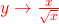in Example 31 is triply flawed: it is meaningless (conceptual demon); the calculation is invalid (computational demon); and the conclusion is wrong (nonlinear demon).

To be fair, it turns out that the -1 in the denominator of the function in Example 31 can be ignored, but not because of the highest powers trick that Cambridge appears to employ. This also means y = √x will be the non-linear asymptote to y = (x + 1)/√(x-1) if we suitably generalise the notion of “polynomial asymptote”.

That’s plenty wrong, of course. But to round off, here are the other problems with the excerpt:

• The “graph of y = f(x)” doesn’t approach anything. It is the function that approaches the asymptote.
• Underlying the punchline, the definition of “non-vertical asymptote” is hopelessly vague and does not remotely mean what Cambridge thinks it means.
• We do not “require √(x – 1) > 0; we require x – 1 > 0.

And, we’re done. Thank Christ.